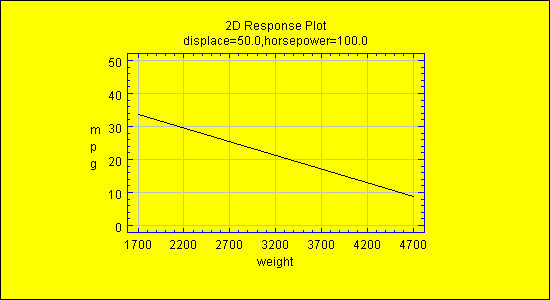# STATBEAN®

Purpose: - creates a 2d plot of the response in a multiple regression model.

Multiple Regression Calculator DataSource: MultipleRegression.Multiple Regression Calculator Read/Write Properties
 Name Type Description Possible Values Default Value holdAt double[nxvars] Values for each x variable to be used in evaluating response (ignored for variable plotted on x axis). Any double values. Means of each variable. xVariableNumber int Variable number in the model for which the component is to be plotted. 0+ 0

Other properties used to create a multiple regression model are inherited from the java.awt.Canvas class and from the general GraphicalStatbean class.

Code Sample - see MultipleRegression.